## Example Questions

2 Next →

### Example Question #1156 : Algebra

Solve: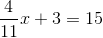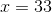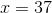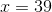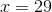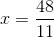Explanation:

We want to isolate the x. First, we take away 3 from both sides. Then we have: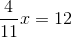To get x by itself, we multiply by the reciprocal on both sides.

Then, we have: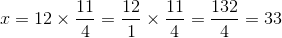### Example Question #1 : How To Solve For A Variable As Part Of A Fraction

If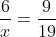, then what is the value of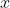?

7/12

none of these

9/114

3/38

38/3

38/3

Explanation:

cross multiply:

(6)(19) = 9x

114=9x

x = 38/3

### Example Question #1 : How To Solve For A Variable As Part Of A Fraction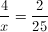Find x.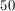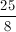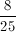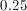NoneExplanation:

Cross multiply: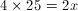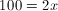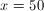### Example Question #2 : How To Solve For A Variable As Part Of A Fraction

The numerator of a fraction is the sum of 4 and 5 times the denominator. If you divide the fraction by 2, the numerator is 3 times the denominator. Find the simplified version of the fraction.Explanation:

Let numerator = N and denominator = D.

According to the first statement,

N = (D x 5) + 4.

According to the second statement, N / 2 = 3 * D.

Let's multiply the second equation by –2 and add itthe first equation:

–N = –6D

+[N = (D x 5) + 4]

=

–6D + (D x 5) + 4 = 0

–1D + 4 = 0

D = 4

Thus, N = 24.

Therefore, N/D = 24/4 = 6.

### Example Question #71 : Algebraic Fractions

Solve for x: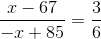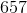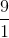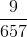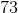Explanation:

In order to solve for x, you must cross multiply the ratio first. They will be equal to each other.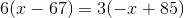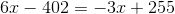, you then can add 3x to both sides and 402 to both sides as well. You are left with: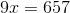divide both sides by 9 to get the final answer: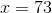2 Next →